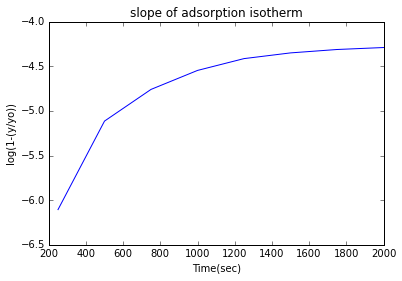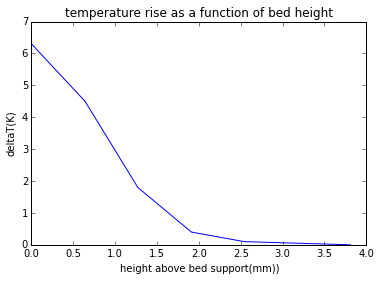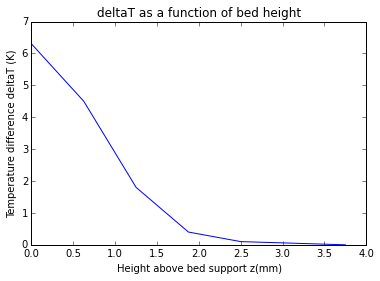# Chapter 6 - FLUIDISATION¶

## Page 298 Example 6.1¶

In :
from math import sqrt
#Calculating minimum fluidisation velocity

#Calculating Galileo number
def Galileo_number():
d = 3*10**(-3)#  #particle size is in meters
p = 1100#       #density of liquid is in kg/m**3
ps = 4200#      #density of spherical particles is in kg/m**3
g = 9.81#       #acceleration due to gravity is in m/sec**2
u = 3*10**(-3)#  #viscosity is in Ns/m**2
Ga = d**3*p*(ps-p)*g/u**2#
return Ga
Ga = Galileo_number()#
print"\nGalileo number = %.3f  *10**5"%(Ga*10**(-5))

#Calculating Re mf
Remf = 25.7*(sqrt(1+5.53*10**(-5)*(1.003*10**5))-1)#
print"\nValue of Remf is %d"%(Remf)#

umf = Remf*(3*10**(-3))/(3*10**(-3)*1100)#
print"\nminimum fluidisation velocity is %.1f mm/sec"%(umf*1000)#

Galileo number = 1.004  *10**5

Value of Remf is 40

minimum fluidisation velocity is 36.4 mm/sec


## Page 299 Example 6.2¶

In :
from math import sqrt,pi
#Calculating voidage by considering eight closely packed spheres of diameter d in a cube of size 2d
print"\n (a)"
def voidage():
d = 1*10**(-4)#    #diameter is in meters
meu = 3*10**(-3)#  #viscosity is in Ns/m**2
ps = 2600#        #density is in kg/m**3
p = 900#         #density is in kg/m**3
e = (8*d**(3)-8*(pi/6)*d**(3))/(8*d**(3))#
return e
e = voidage()#
print"\nvoidage = %.2f"%(e)#

#Calculating minimum fluidisation mass flow rate

def min_fluidis_vel():
e = voidage()#
d = 1*10**(-4)#    #diameter is in meters
meu = 3*10**(-3)#  #viscosity is in Ns/m**2
ps = 2600#        #density is in kg/m**3
p = 900#         #density is in kg/m**3
g = 9.81#         #acceleration due to gravity is in m/sec**2
Gmf = 0.0055*(e)**(3)/(1-e)*(d**2)*p*(ps-p)*g/meu#
return Gmf
Gmf = min_fluidis_vel()#
print"\nminimum fluidisation velocity is %.3f kg/m**2sec"%(Gmf)#

print"\n (b)"
def terminal_velocity():
e = voidage()#
d = 1*10**(-4)#    #diameter is in meters
meu = 3*10**(-3)#  #viscosity is in Ns/m**2
ps = 2600#        #density is in kg/m**3
p = 900#         #density is in kg/m**3
g = 9.81#         #acceleration due to gravity is in m/sec**2
u = d**(2)*g*(ps-p)/(18*meu)#
return u
print"\nterminal velocity is %.4fm/sec"%(terminal_velocity())#

#Reynolds no for this Terminal velocity is
Re = (10**(-4)*0.0031*900)/(3*10**(-3))#
print"\nReynlds no =%.3f"%(Re)#
print"\nThe required mass flow rate is %.2f kg/m**2sec"%(terminal_velocity()*900)#


 (a)

voidage = 0.48

minimum fluidisation velocity is 0.057 kg/m**2sec

(b)

terminal velocity is 0.0031m/sec

Reynlds no =0.093

The required mass flow rate is 2.78 kg/m**2sec


# Page 305 Example 6.3¶

In :
from sympy import symbols,solve
# to calculate voidage of the bed

def Galileo_number():
d = 4*10**(-3)#  #particle size is in meters
p = 1000#       #density of water is in kg/m**3
ps = 2500#      #density of glass is in kg/m**3
g = 9.81#       #acceleration due to gravity is in m/sec**2
u = 1*10**(-3)#  #viscosity is in Ns/m**2
Ga = d**3*p*(ps-p)*g/u**2#
return Ga

print"\nGalileo number = %.2f*10**5"%(Galileo_number()*10**(-5))#

def Reynolds_no():
Ga = Galileo_number()#
Re = (2.33*Ga**(0.018)-1.53*Ga**(-0.016))**(13.3)#
return Re
print"\n The Reynolds no is %d"%(Reynolds_no())#
v = Reynolds_no()*(1*10**(-3))/(0.004*1000)#
print"\nvelocity = %.2f m/sec"%(v)#

n = symbols('n')
z = solve((4.8-n)-0.043*(Galileo_number())**(0.57)*(n-2.4))
print"\nvalue of n is %.2f"%(z)#

#voidage at a velocity is 0.25m/sec
e=0.1#
while 1:
enew = e -((0.25/0.45)-e**(2.42))/(-2.42*e**1.42)#
if (enew == e):
print"\nVoidage is %.3f"%(e)#
break#

e=enew#

Galileo number = 9.42*10**5

The Reynolds no is 1798

velocity = 0.45 m/sec

value of n is 2.42

Voidage is 0.784


## Page 345 Example 6.4¶

In :
from numpy import arange,log
from sympy import symbols,solve
%matplotlib inline
from matplotlib.pyplot import plot,xlabel,ylabel,title,show
t = arange(250,2001,250) # #time is in secs
y = [0.00223, 0.00601, 0.00857, 0.0106, 0.0121, 0.0129, 0.0134 ,0.0137]

i =0#
yo = 0.01442#
z=[];

x=[]
while i<8:
z.append(y[(i)]/yo)

x.append(log(y[(i)]))
i=i+1#

xlabel("Time(sec)")
ylabel("log(1-(y/yo))")
plot(t,x)
show()
print"\nFrom the graph the value of slope is %.3f  "%(-0.00167)#
Gm = 0.679*10**(-6)#  #units are in kmol/sec
W = 4.66#            #units are in gram
b = symbols('b')
s = solve(-0.00167*4.66*b+0.679*10**(-6))
print"\n b = %.4f kmol/kg"%(s*10**3)#0From the graph the value of slope is -0.002

b = 0.0873 kmol/kg


## Page 349 Example 6.5¶

In :
from numpy import arange,log,pi
from sympy import symbols,solve
%matplotlib inline
from matplotlib.pyplot import plot,xlabel,ylabel,title,show

gas_flow_rate =0.2#       #units are in kg/m**2
c = 0.88#                 #specific heat capacity of air is kj/kg K
viscosity = 0.015*10**(-3)##viscosity is in Ns/m**2
d = 0.25*10**(-3)#         #particle size is in meters
k = 0.03#                 #thermal conductivity is in W/m K
e = 0.57#                 #e is voidage

T = [339.5, 337.7, 335.0, 333.6, 333.3, 333.2]# #temperature is in kelvins
deltaT=[]
for t in T:
deltaT.append(t - 333.2)
h = [0, 0.64, 1.27, 1.91, 2.54, 3.81]#
title("temperature rise as a function of bed height")
xlabel("height above bed support(mm))")
ylabel("deltaT(K)")
plot(h,deltaT)
show()

#Area under the curve gives the value of  the heat teansfer integral =8.82mm K

q = 0.2*0.88*(339.5-332.2)#
print"\n heat transferred = %.2f kw/m**2 of bed cross sectional area"%(q)#

#Assuming 1m**3 volume
Vp = (1-0.57)#       #Volume of particles is in m**3
print"\n Volume of particles is %.2f m**3"%(Vp)
v1 = (pi/6)*d**3#    #Volume of 1 particle in m**3
print"\n Volume of 1 particle is %.2f*10**(-12) m**3"%(v1*10**(12))#
print"\n number of particles is %.2f*10**(10)"%(Vp/v1*10**(-10))#

x =symbols('x')#
h = solve(1100 - x*(1.03*10**4)*(8.82*10**(-3)))
print"\n heat transfer coefficient = %.1f W/m**2"%(h)#

#Nu = 0.11Re**(1.28)
Re = (0.2*0.25*10**(-3))/(0.015*10**(-3))#
h1 = 0.11*(Re)**(1.28)*k/d#
print"\n h = %.1f W/m**2 K"%(h1)#heat transferred = 1.28 kw/m**2 of bed cross sectional area

Volume of particles is 0.43 m**3

Volume of 1 particle is 8.18*10**(-12) m**3

number of particles is 5.26*10**(10)

heat transfer coefficient = 12.1 W/m**2

h = 61.6 W/m**2 K


## Page 350 Example 6.6¶

In :
from __future__ import division
from sympy import symbols,solve
%matplotlib inline
from matplotlib.pyplot import plot,xlabel,ylabel,title,show

cp = 0.85#                              #specific heat capacity of the air
h = [0, 0.625, 1.25, 1.875, 2.5, 3.75]#                       #height in mm
T=[339.5, 337.7, 335.0 ,333.6 ,333.3, 333.2]##temperature in K

deltaT=[]
for t in T:
deltaT.append(t - 333.2)
#temperature difference in kelvins
plot(h,deltaT)
title("deltaT as a function of bed height")
xlabel("Height above bed support z(mm)")
ylabel("Temperature difference deltaT (K)")
show()
#From the plot area under the curve is 6.31 K mm
sp = (6/(0.25*10**(-3)))*(0.5)#  #sp is surface area per unit volume in m**2/m**3
G = 0.2#                        #in kg/m**2sec
Cp = 850#                       #Cp is in J/kg K
h1 = symbols('h1')
s = solve(0.2*850*6.3-h1*1.2*10**(4)*6.31*10**(-3))
print" \n Coefficient for heat transfer between the gas and the particles= %.1fW/m**2 K"%(s)#

print"\n Let the evaporation rate be 0.1 kg/sec at a temp difference = 50 degK"
mdot = 0.1#         #evaporation rate is 0.1 kg/sec
Latent_heat = 2.6*10**(6)#
print"\n The heat flow = %.1f*10**5 W"%(mdot*Latent_heat*10**(-5))#
A=(2.6*10**5)/(14.1*50)#
print"\n the effective area of the bed A = %d m**2"%(A)#
print"\n The surface area of the bed = %d m**2"%(0.1*1.2*10**4)#
print"\n hence the fraction of the bed = %.2f"%(369/1200)#Coefficient for heat transfer between the gas and the particles= 14.1W/m**2 K

Let the evaporation rate be 0.1 kg/sec at a temp difference = 50 degK

The heat flow = 2.6*10**5 W

the effective area of the bed A = 368 m**2

The surface area of the bed = 1200 m**2

hence the fraction of the bed = 0.31